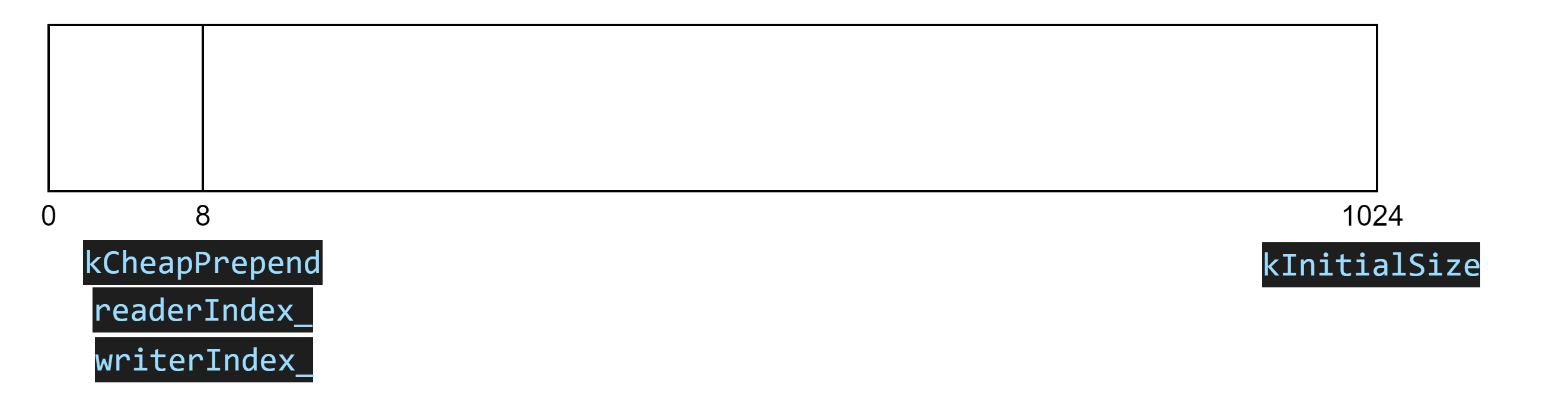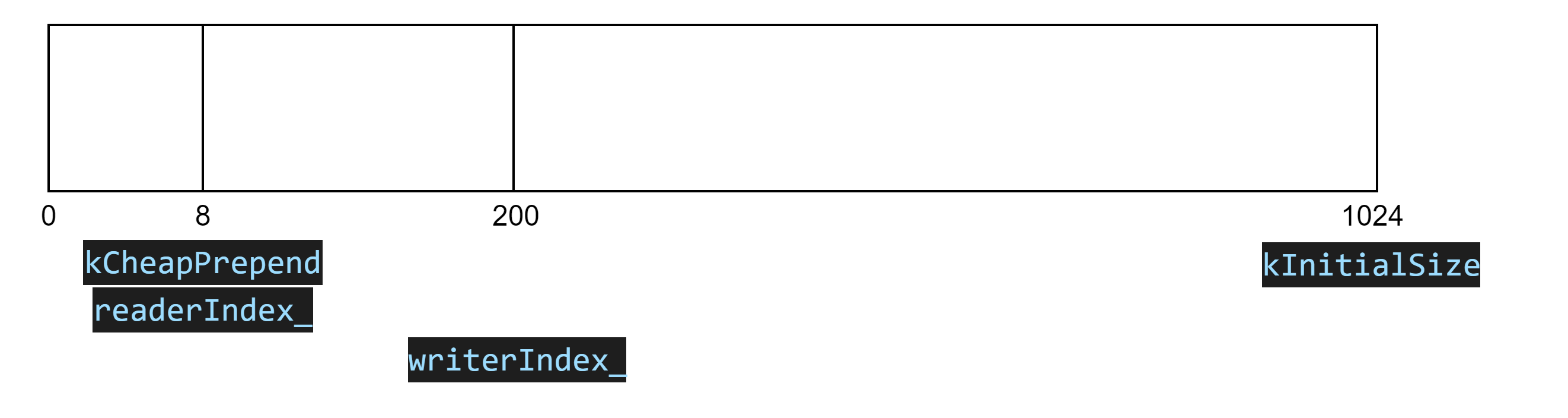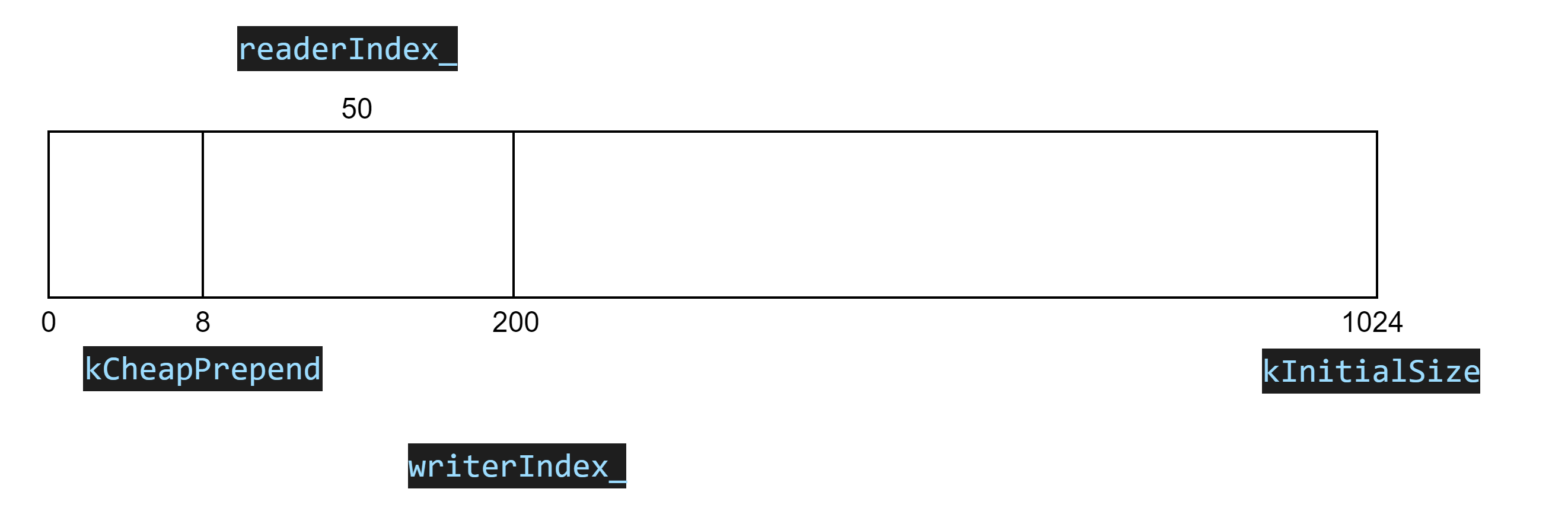muduo源码分析之Buffer• 累计撰写 36 篇文章
• 累计收到 19 条评论

# muduo源码分析之Buffer2022-05-08 / 0 评论 / 88 阅读 / 正在检测是否收录...``````    // 把onMessage函数上报的buffer内容转为string
std::string retrieveAllAsString()
{
}

// 可读的数据 就是存放的是即发送的数据
{
}

std::string retrieveAsString(size_t len)
{
// 从可读数据开始位置，长度为len的char构造为一个string
std::string result(peek(), len);
retrieve(len); // 上面一句把缓冲区中可读的数据，已经读取出来，这里肯定要对缓冲区进行复位操作
return result;
}

// 将缓冲区len的长度进行复位
void retrieve(size_t len)
{
// 表示还没有读完数据
{
}
{
retrieveAll();
}
}``````

``````ssize_t Buffer::readFd(int fd, int* saveErrno)
{
char extrabuf = {0}; // 栈上的内存空间  64K

struct iovec vec;

// 这是buffer可写的数据
const size_t writable = writableBytes();
vec.iov_base = begin() + writerIndex_;
vec.iov_len = writable;

vec.iov_base = extrabuf;
vec.iov_len = sizeof extrabuf;

const int iovcnt = (writable < sizeof extrabuf) ? 2 : 1;
const ssize_t n = ::readv(fd, vec, iovcnt);
if (n < 0)
{
*saveErrno = errno;
}
else if (n <= writable) // Buffer的可写缓冲区已经够存储读出来的数据了
{
writerIndex_ += n;
}
// extrabuf 也写了数据
else
{
writerIndex_ = buffer_.size();
append(extrabuf, n - writable);  // writerIndex_开始写 n - writable大小的数据
}

return n;
}``````

``````    // 要写len长度的数据
void ensureWriteableBytes(size_t len)
{
if (writableBytes() < len)
{
makeSpace(len); // 扩容函数
}
}

// 向缓冲区添加数据
void append(const char *data, size_t len)
{
ensureWriteableBytes(len);
std::copy(data, data+len, beginWrite());
writerIndex_ += len;
}````````````    void makeSpace(size_t len)
{
if (writableBytes() + prependableBytes() < len + kCheapPrepend)
{
buffer_.resize(writerIndex_ + len);
}
else
{
begin() + writerIndex_,
begin() + kCheapPrepend);
}
}``````

``````// 通过fd发送数据
ssize_t Buffer::writeFd(int fd, int* saveErrno)
{
ssize_t n = ::write(fd, peek(), readableBytes());
if (n < 0)
{
*saveErrno = errno;
}
return n;
}``````
0